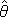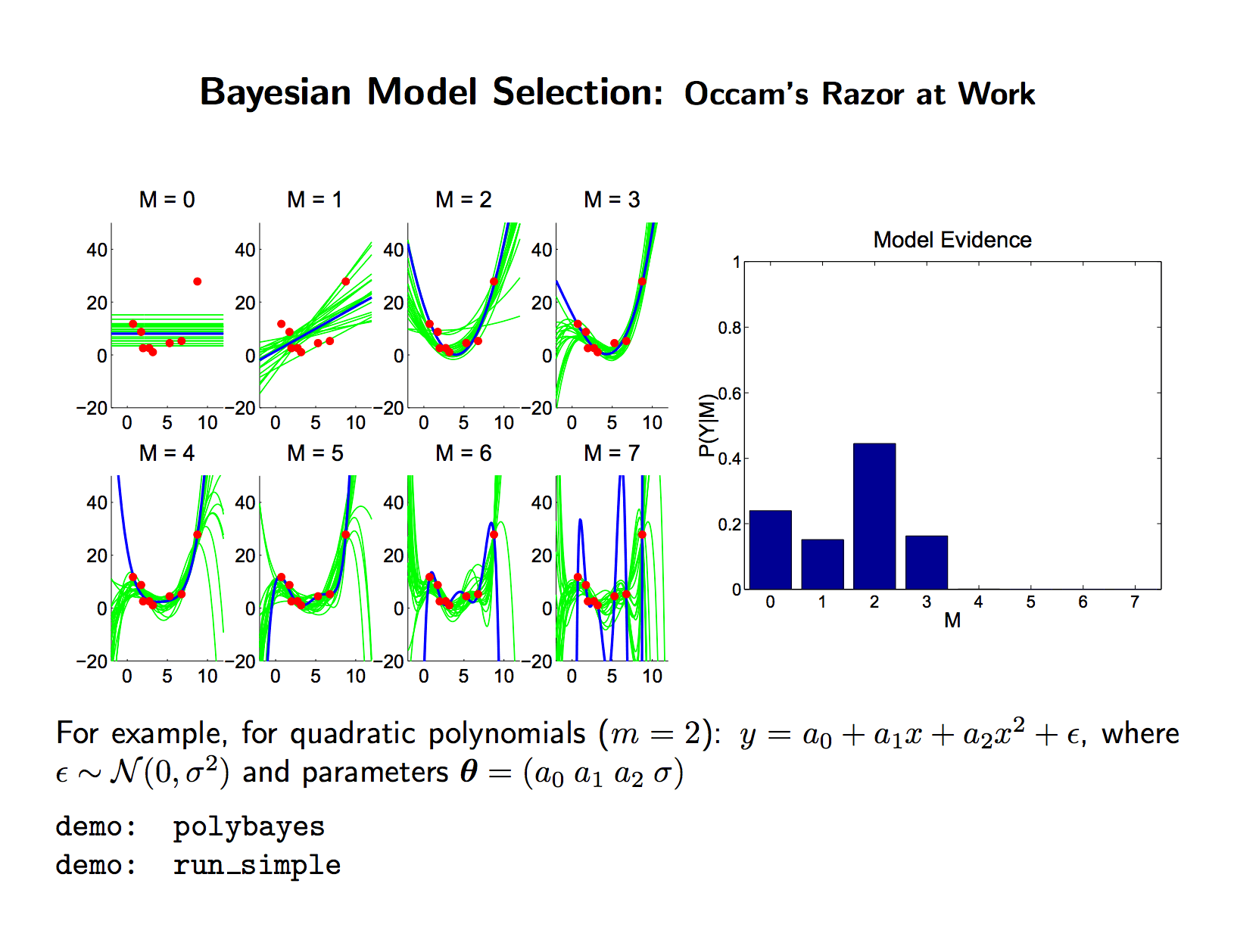# Bayesian Occam’s Razor

A couple of days ago I posted a question that has been bugging me; namely, does Bayes’ overfit, and if not, why not?

Today I post the solution!

There are two parts: first, explaining where my initial argument against Bayes went wrong, and second, describing the Bayesian Occam’s Razor, the key to understanding how a Bayesian deals with overfitting.

# Part 1: Why I was wrong

Here’s the argument I wrote initially:

1. Overfitting arises from an excessive focus on accommodation. (If your only epistemic priority is accommodating the data you receive, then you will over-accommodate the data, by fitting the noise in the data instead of just the underlying trend.)
2. We can deal with overfitting by optimizing for other epistemic virtues like simplicity, predictive accuracy, or some measure of distance to truth. (For example, minimum description length and maximum entropy optimize for simplicity, and cross validation optimizes for predictive accuracy).
3. Bayesianism is an epistemological procedure that has two steps, setting of priors and updating those priors.
4. Updating of priors is done via Bayes’ rule, which rewards theories according to how well they accommodate their data (creating the potential for overfitting).
5. Bayesian priors can be set in ways that optimize for other epistemic virtues, like simplicity or humility.
6. In the limit of infinite evidence, differences in priors between empirically distinguishable theories are washed away.
7. Thus, in the limit, Bayesianism becomes a primarily accommodating procedure, as the strength of the evidential update swamps your initial differences in priors.

Here’s a more formal version of the argument:

1. The relative probabilities of two model given data is calculated by Bayes’ rule:
P(M | D) / P(M’ | D)  = P(M) / P(M’)・P(D | M) / P(D | M’)
2. If M overfits the data and M’ does not, then as the size of the data set |D| goes to infinity, the likelihood factor P(D | M) / P(D | M’) goes to infinity.
3. Thus the posterior probability P(M | D) should go to 1 for the model that most drastically overfits the data.

This argument is wrong for a couple of reasons. For one, the argument assumes that as the size of the data set grows, the model stays the same. But this is very much not going to be true in general. The task of overfitting gets harder and harder as the number of data points go up. It’s not that there’s no longer noise in the data; it’s that the signal becomes more and more powerful.

A perfect polynomial fit on 100 data points must have, at the worst, 100 parameters. On 1000 data points: 1000 parameters. Etc. In general, as you add more data points, a model that was initially overfitting (e.g. the 100-parameter distribution) will find that it is harder and harder to ignore the signal for the noise, and the next best overfitting model will have more parameters (e.g. the 1000-parameter distribution).

But now we have a very natural solution to the problem we started with! It is true that as the number of data points increases, the evidential support for the model that overfits the data will get larger and larger. It’s also true is that the number of parameters required to overfit the data will grow as well. So if your prior in a model is a decreasing function of the number of parameters in the model, then you can in principle find a perfect balance and avoid overfitting. This perfect balance would be characterized by the following: each time you increase the number of parameters, the prior should decrease by an amount proportional to how much more you get rewarded by overfitting the data with the extra parameters.

How do we find this prior in practice? Beats me… I’d be curious to know, myself.

But what’s most interesting to me is that to solve overfitting as a Bayesian, you don’t even need the priors; the solution comes from the evidential update! It turns out that in fact, the likelihood function for updating credences in a model given data automatically incorporates in model overparameterization. Which brings us to part 2!

# Part 2: Bayesian Occam’s Razor

That last sentence bears repeating. In reality, although priors can play some role by manually penalizing models with high overfitting potential, the true source of the Bayesian Occam’s razor comes from the evidential update. What we’ll find by the end of this post is that models that overfit don’t actually get a stronger evidential update than models that don’t.

You might wonder how this is possible. Isn’t it practically the definition of overfitting that it is an enhancement of the strength of an evidential update through fitting to noise in the data?

Sort of. It is super important to keep in mind the distinction between a model and a distribution. A distribution is a single probability function over your possible observable data. A model is a set of distributions, characterized by a set of parameters. When we say that some models have the potential to overfit a set of data, what we are really saying is that some models contain distributions that overfit the data.

Why is this important? Because assessing the posterior probability of the model is not the same as assessing the posterior probability of the overfitting distribution within the model! Here’s Bayes’ rule, applied to the model and to the overfitting distribution:

(1) P(M | D) = P(M)・P(D | M) / P(D)

(2) P(| D) = P()・P(D |) / P(D)

It’s clear how to evaluate equation (2). You have some prior probability assigned to, you know how to assess the likelihood function P(D |), and P(D) is an integral that is in principle do-able. In addition, equation (2) has the scary feature we’ve been talking about: the likelihood function P(D |) is really really large if our parameteroverfits the data, potentially large enough to swamp the priors and screw up our Bayesian calculus.

But what we’re really interested in evaluating is not equation (2), but equation (1)! This is, after all, model selection; we are in the end trying to assess the quality of different models, not individual distributions.

So how do we evaluate (1)? The key term is P(D | M); your prior over the models and the data you receive are not too important for the moment. What is P(D | M)? This question does not actually have an obvious answer… M is a model, a set of distributions, not a single distribution. If we were looking at one distribution, it would be easy to assess the likelihood of the data given that distribution.

So what does P(D | M) really mean?

It represents the average probability of the data, given the model. It’s as if you were to draw a distribution at random from your model, and see how well it fits the data. More precisely, you draw a distribution from your model, according to your prior distribution over the distributions in the model.

That was a mouthful. But the basic idea is simple; a model is an infinite set of distributions, each corresponding to a particular set of values for the parameters that define the model. You have a prior distribution over these values for the parameters, and you use this prior distribution to “randomly” select a distribution in your model. You then assess the probability of the data given that distribution, and voila, you have your likelihood function.

In other words…

P(D | M) = ∫ P(D | θ) P(θ | M) dθ

Now, an overfitting model has a massive space of parameters, and in some small region of this space contains distributions that fit the data really well. On the other hand, a simple model that generalizes well has a small space of parameters, and a region of this space contains distributions that fit the data well (though not as well as the overfitter).

So on average, you are much less likely to select the optimal distribution in the overfitting model than in the generalizable model. Why? Because the space of parameters you must search through to find it is so much larger!

True, when you do select the optimal distribution in the overfitting model, you get rewarded with a better fit to the data than you could have gotten from the nice model. But the balance, in the end, pushes you towards simpler and more general models.

This is the Bayesian Occam’s Razor! Models that are underparameterized do poorly on average, because they just can’t fit the data at all. Models that are overparametrized do poorly on average, because the subset of the parameter space that fits the data well is so tiny compared to the volume of the parameter space as a whole. And the models that strike the perfect balance are those that have enough parameters to fit the data well, but not too many as to excessively bloat the parameter space.

Here are some lecture slides from these great notes that have some helpful visualizations:Recapping in a few sentences: Simpler models are promoted, simply because they do well on average. And evidential support for a model comes down to the performance on average, not optimal performance. The likelihood in question is not P(data | best distribution in model), it’s P(data | average distribution in model). So overfitting models actually don’t get as much evidential support from data when assessing the model quality as a whole!

Ain’t that cool??